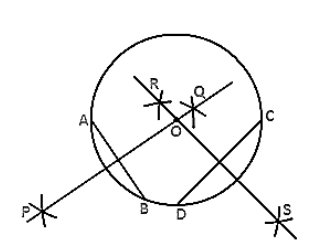Deepak Scored 45->99%ile with Bounce Back Crack Course. You can do it too!

# Draw a circle with center at point O. Draw its two chords AB and CD such that AB is not parallel to CD.

Question:

Draw a circle with center at point O. Draw its two chords AB and CD such that AB is not parallel to CD. Draw the perpendicular bisectors of AB and CD. At what point do they intersect?

Solution:

Steps of construction:

1. Keeping O as the centre and any radius draw a circle.

2. Draw two chords AB and CD.

3. Keeping A as center and radius more than half of AB draw arcs, one on each side of AB.

4. Keeping B as center and the same radius draw arcs cutting the previous arcs at point P and Q respectively.

5. Join the points P and Q.

6. Keeping D as center and radius more than half of DC draw arcs, one on each side of DC.

7. Keeping C as center and the same radius draw arcs cutting the previous arcs at points R and S respectively.

8. Join the points R and S.

Both the perpendicular bisectors PQ and RS intersect each other at the center O of the circle.